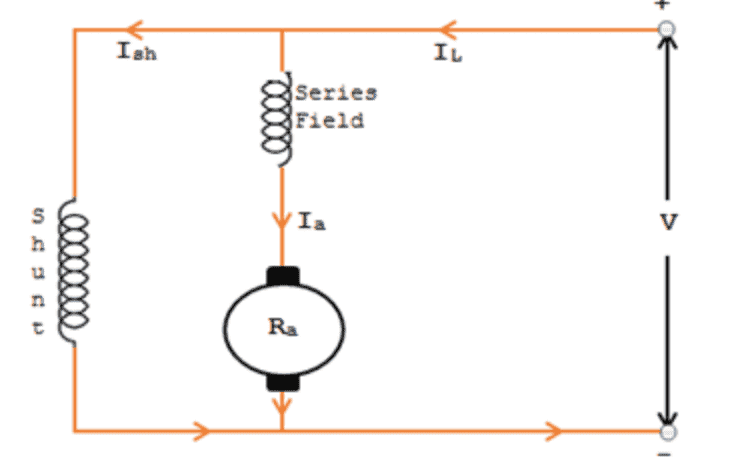# Compound wound D.C. motor

• Engineering
• niteOwl

#### niteOwl

Homework Statement
A 500 W, 200 V compound motor has two field windings, one has a resistance of
0.03Ω and the other a resistance of 50 Ω.
i) If the armature resistance is 0.15 Ω, calculate the emf when it draws an
armature current of 5A whilst running at 3000 rpm.
ii) When the load increases so that the motor draws a current of 10A, what is
the new speed of the machine? (assume the field shunt current does not
change – just the series field)
Relevant Equations
...
I am assuming the motor is cumulative compound wound and that it is long shunt. I also assume that the shunt field resistance ##R_{shunt}## = 50Ω, while the series field resistance is ##R_{series}## = 0.03Ω.

i) calculate EMF with armature current ##I_{a}## = 5A and armature resistance ##R_{a}## = 0.15Ω.
For a motor with long shunt compound winding, the terminal voltage is

##V_{T} = E + I_{a}(R_{a} + R_{series})##, where E is emf. Therefore
$$E = V_{T} - I_{a}(R_{a} + R_{series})$$
E = 200V - 5A * (0.15Ω + 0.03Ω) = 199.1V
E = 199.1V

ii) calculate motor speed ##n## when ##I_{a}## = 10A

This is where I get really stuck. We're told the shunt field remains constant and only the series field changes with the increased ##I_{a}##.

With indexes 1 and 2 referring to intial current and increased current respectively, I know that in general:
$$\frac{n_{2}}{n_{1}} = \frac{E_{2}}{E_{1}}\frac{\Phi_{1}}{\Phi_{2}}$$
If we had either a shunt or series wound machine, the unknown ##\Phi## would cancel out, but for our compound wound machine
$$\Phi_{2} = \Phi_{1} + \Delta\Phi = \Phi_{shunt} + \Phi_{series} + \Delta\Phi_{series}$$
with ##\Phi \propto I## this becomes
$$\Phi_{2} = \Phi_{shunt} + 3\Phi_{series}$$

This means we can't cancel the magnetic flux.

Last edited:

$$\frac{n_{2}}{n_{1}} = \frac{E_{2}}{E_{1}}\frac{\Phi_{1}}{\Phi_{2}} = \frac{E_{2}}{E_{1}}\frac{I_{1}}{I_{2}}$$
Now
$$I_{2} = I_{1} + \Delta I = I_{1} +(I_{a, 2} - I_{a, 1}) = I_{1} + (10A - 5A) = I_{1} + 5A$$
where ##I_{a}## is armature current. Therefore
$$\frac{n_{2}}{n_{1}} = \frac{E_{2}}{E_{1}}\frac{I_{1}}{I_{1} + 5A}$$
Now as the initial net current ##I_{1} = I_{f} + I_{a, 1}## where the shunt field current ##I_{f}## is constant.
Therefore
$$I_{1} = I_{f} + I_{a, 1} = \frac{200V}{50\Omega} + 5A = 9A$$
and
$$\frac{n_{2}}{n_{1}} =\frac{9}{14} \frac{E_{2}}{E_{1}} =\frac{9}{14} \frac{200V - 10A*0.18\Omega}{200V - 5A*0.18\Omega}$$
and from here it is simple to solve for ##n_{2}##

Is this correct? One thing that bothers me about this question is if you divide the output power by the terminal voltage, you get an armature current that is below both of the values given in the question.

Hi,

Sorry if this is late. Before I write out a response, can I just confirm my understanding of this 'compound' motor set-up (haven't come across that term before when I studied DC motors).

1) Is this diagram below consistent with how you visualize the motor?
From the equations for part (a), it seems so.2) It has been a while since I studied this topic, so for part (b) when it says 'draws a current', are we sure that refers to the armature current (instead of the load current)?

3) For this set-up of a compound motor, is the motor constant still ## k = k' \cdot I_{field}##?

Thanks

Hi,
Yes the diagram is correct, at least I assume so, as there are two possible types of configuration, long shunt and short shunt. The question does not indicate which type but I assume it is long shunt, seems to be most common, and is exactly like your diagram. (In case of short shunt, the series coil is in series with both the shunt and the armature, which together form a parallel network. See here)

Yes, again. The 10 amps drawn by the motor refer to the current in the armature ##I_{a}##. This was another point of confusion to me because, $$I_{a} = P / V_{T}$$, where ##P## is rated power and ##V_{T}## is terminal voltage. Given ##P = 500W## and ##V_{T} = 200V## as stated in the question, this value of 10A is 4 times nominal current. I'm just getting to grips with this subject, but given a constant supply voltage, wouldn't this push excessive power through the motor?

I am not familiar with the motor constant unfortunately, it didn't come up so far in the course I'm studying.